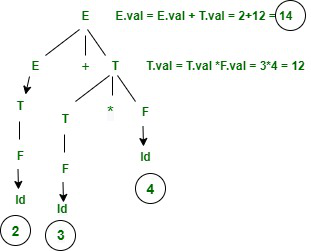# Application of Syntax Directed Translation

• Difficulty Level : Medium
• Last Updated : 21 Aug, 2020

In this article, we are going to cover the application of Syntax Directed Translation where we will also see real example and how can solve the problem with these applications. let’s discuss one by one.

Pre-requisite : Introduction to Syntax Directed Translation

Syntax Directed Translation :
It is used for semantic analysis and SDT is basically used to construct the parse tree with Grammar and Semantic action. In Grammar, need to decide who has the highest priority will be done first and In semantic action, will decide what type of action done by grammar.

Example :

```SDT = Grammar+Semantic Action
Grammar = E -> E1+E2
Semantic action= if (E1.type != E2.type) then print "type mismatching"```

Application of Syntax Directed Translation :

• SDT is used for Executing Arithmetic Expression.
• In the conversion from infix to postfix expression.
• In the conversion from infix to prefix expression.
• It is also used for Binary to decimal conversion.
• In counting number of Reduction.
• In creating a Syntax tree.
• SDT is used to generate intermediate code.
• In storing information into symbol table.
• SDT is commonly used for type checking also.

Example :
Here, we are going to cover an example of application of SDT for better understanding the SDT application uses. let’s consider an example of arithmetic expression and then you will see how SDT will be constructed.

Let’s consider Arithmetic Expression is given.

```Input : 2+3*4
output: 14```

SDT for the above example.SDT for 2+3*4

Semantic Action is given as following.

```E -> E+T  { E.val = E.val + T.val then print (E.val)}
|T   { E.val = T.val}
T -> T*F  { T.val = T.val * F.val}
|F   { T.val = F.val}
F -> Id   {F.val = id}```
My Personal Notes arrow_drop_up